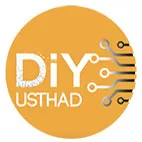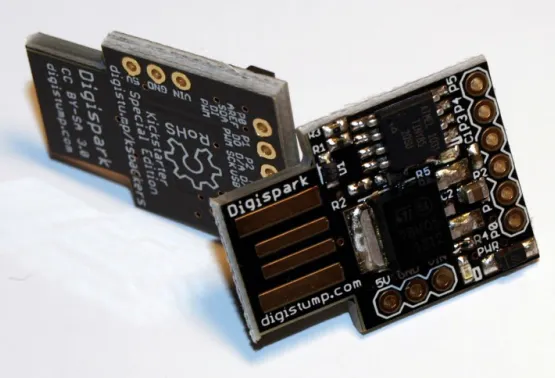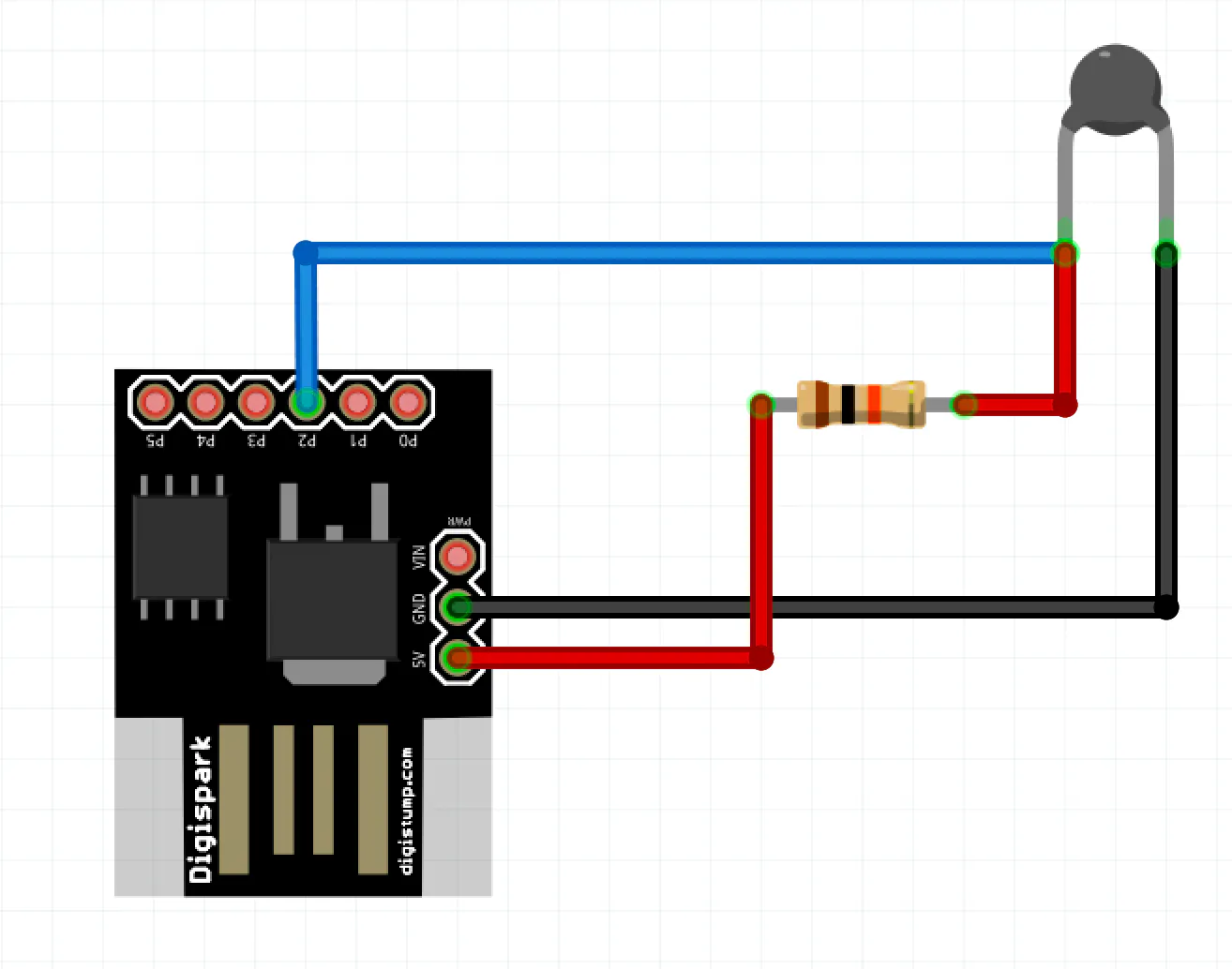# Phone Thermometer Using Digispark Attiny85

In this tutorial, we are going to build a simple OTG Phone Thermometer using Digispark ATtiny85 that can connect to our mobile phone.

BeginnerFull instructions provided2,242

## Things used in this project

### Hardware componentsDigiSpark
×1Resistor 10k ohm
×1
 10k Precision thermistor
×1
×1

### Software apps and online services

 Serial USB Terminal

## Schematics

### Circuit## Code

### Arduino Code

Arduino
```#include <DigiCDC.h>

// the value of the 'other' resistor
#define SERIESRESISTOR 10000
// What pin to connect the sensor to
#define THERMISTORPIN A1
#define THERMISTORNOMINAL 10000
// temp. for nominal resistance (almost always 25 C)
#define TEMPERATURENOMINAL 25
// how many samples to take and average, more takes longer
// but is more 'smooth'
#define NUMSAMPLES 5
// The beta coefficient of the thermistor (usually 3000-4000)
#define BCOEFFICIENT 3950

void setup() {
SerialUSB.begin();
}

// the loop routine runs over and over again forever:
void loop()
{
if (SerialUSB.available())
{
uint8_t i;
float average;

// convert the value to resistance
average = 1023 / average - 1;
average = SERIESRESISTOR / average;
//SerialUSB.print("Thermistor resistance ");
//SerialUSB.println(average);

float steinhart;
float presteinhart;
steinhart = average / THERMISTORNOMINAL;     // (R/Ro)
steinhart = log(steinhart);                  // ln(R/Ro)
steinhart /= BCOEFFICIENT;                   // 1/B * ln(R/Ro)
steinhart += 1.0 / (TEMPERATURENOMINAL + 273.15); // + (1/To)
steinhart = 1.0 / steinhart;                 // Invert
steinhart -= 273.15;                         // convert absolute temp to C

if (int(presteinhart) != int(steinhart))
{
SerialUSB.print("Temperature ");
SerialUSB.print(steinhart);
SerialUSB.println(" *C");
presteinhart = steinhart;
}
SerialUSB.delay(1000);
}
}
```